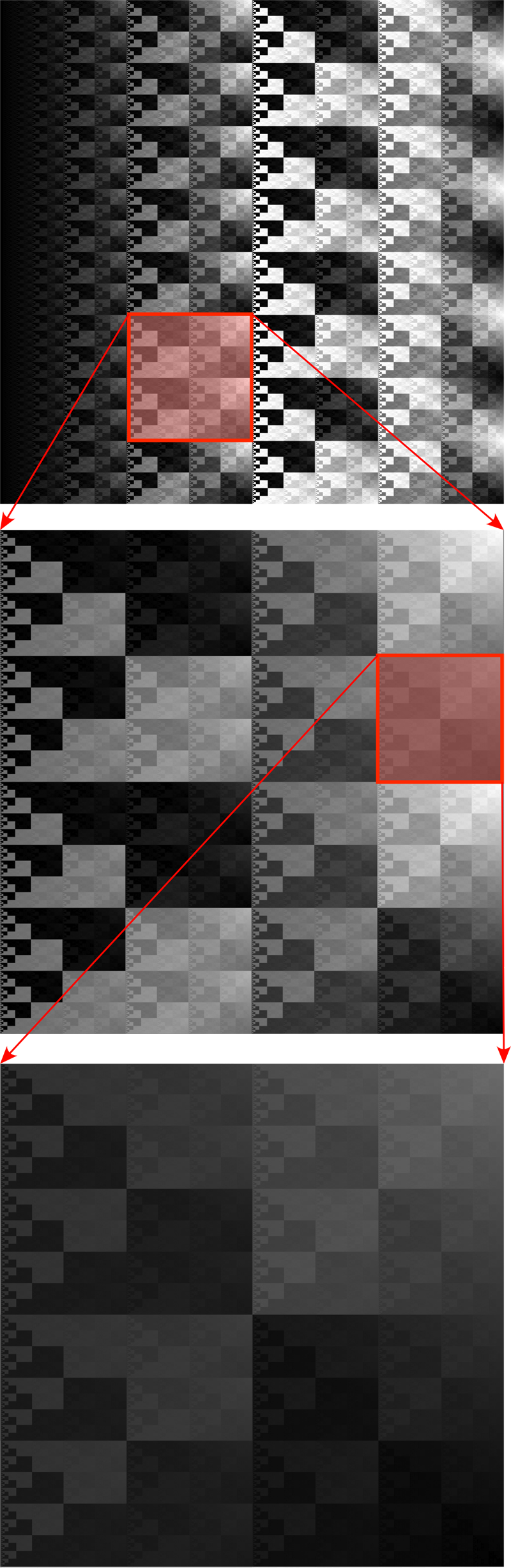# Square tile fractal

Written by Paul Bourke
January 2013

Based upon work by Santiago Zubieta

The tiles below are based upon the value of the following expression modulo some integer (M) which turns out to be the repeating width of the tile.

i & (j - 2 * (i^j) + j) & i

Where (i,j) is the index of the pixel in question, and "&" and "^" are bit-wise AND and XOR respectively. If M is a power of 2 then the tile is complete.Original code snippet from Santiago Zubieta follows:

```for (int i=0; i<width; ++i) {
for (int j=0; j<height; ++j) {
int x = i & (j - 2*(i^j) + j) & i;
x %= 256;
x = Math.abs(x);
g.setColor(new Color(x,x,x));
g.drawRect(i, j, 1, 1);
}
}```The self-similarity across scales can be observed by producing a single tile at high resolution, in this case 16384 pixels (top image below). Each subsequent image is a 4x zoom into the region shown.